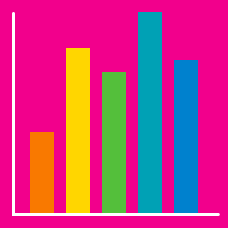Probability

# Two Way Tables

The following two-way table represents a class of students:

$\begin{array}{l|cc} & \text{Boys} & \text{Girls} \\ \hline \text{Tall} & 7 & 4 \\ \text{Short} & 3 & 7 \end{array}$

How many tall boys are in the class?

The following two-way table represents the cars in a small parking lot one morning,

$\begin{array}{l|ccc} & \text{White} & \text{Red} & \text{Blue} \\ \hline \text{Small} & 7 & 6 & 9 \\ \text{Large} & 6 & 5 & 7 \end{array}$

If I see a person walking toward the lot and assume they will get into a random car that is parked there, what is the probability they will choose a large, red car?

The following two-way table represents the cars in a small parking lot one morning,

$\begin{array}{l|ccc} & \text{White} & \text{Red} & \text{Blue} \\ \hline \text{Small} & 7 & 6 & 9 \\ \text{Large} & 6 & 5 & 7 \end{array}$

If I turn away for a second, and then see a blue car leave the parking lot (the time interval was too short for multiple cars to leave, and we can assume any car from the parking lot was just as likely to leave), what is the probability that it was a small car?

The following two-way table represents a class of 90 students that has more girls than boys:

$\begin{array}{l|cc} & \text{Boys} & \text{Girls} \\ \hline \text{Passed} & 25 & & \\ \text{Failed} & & 10 \\ \end{array}$

However, some of its entries have been erased and are missing. If we know that in this class, a randomly chosen boy and a randomly chosen girl both have the same probability of passing, how many boys who failed are there?

The following two-way table represents the animals seen in a park one evening,

$\begin{array}{l|cc} & \text{Dogs} & \text{Cats} \\ \hline \text{Big} & 8 & 4 \\ \text{Small} & 7 & 26 \end{array}$

At this park, how many times larger is the probability that a random dog is big than the probability that a random cat is big?

×